#Algebra 2 Worksheets

## Conic Sections Worksheets

Here is a graphic preview for all of the Conic Sections Worksheets. You can select different variables to customize these Conic Sections Worksheets for your needs. These Conic Sections Worksheets are randomly created and will never repeat so you have an endless supply of quality Conic Sections Worksheets to use in the classroom or at home.

Our Conic Sections Worksheets are free to download, easy to use, and very flexible.

These Conic Sections Worksheets are a good resource for students in the 9th Grade through the 12th Grade.

Click here for a Detailed Description of all the Conic Sections Worksheets.

## Quick Link for All Conic Sections Worksheets

Click the image to be taken to that Conic Sections Worksheets.

##### Propertiesof CirclesAlgebra 2 Worksheets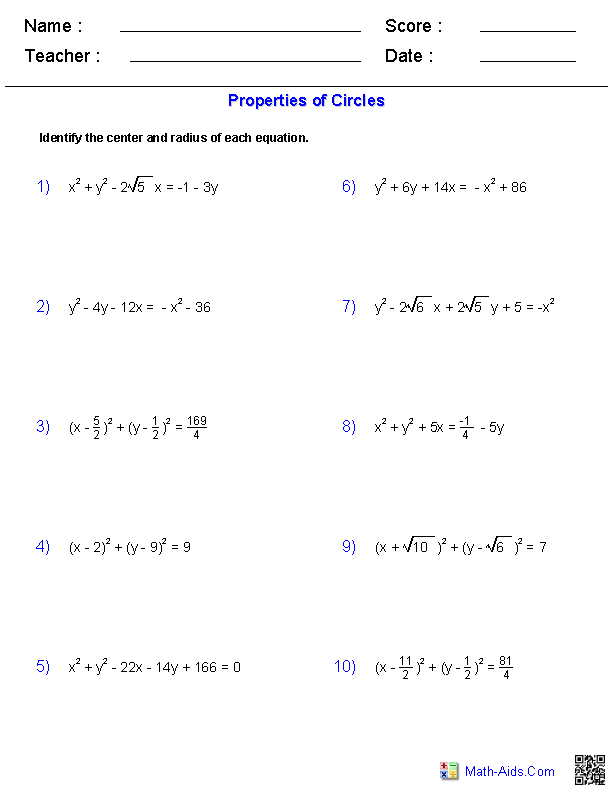##### Writing Equationsof CirclesAlgebra 2 Worksheets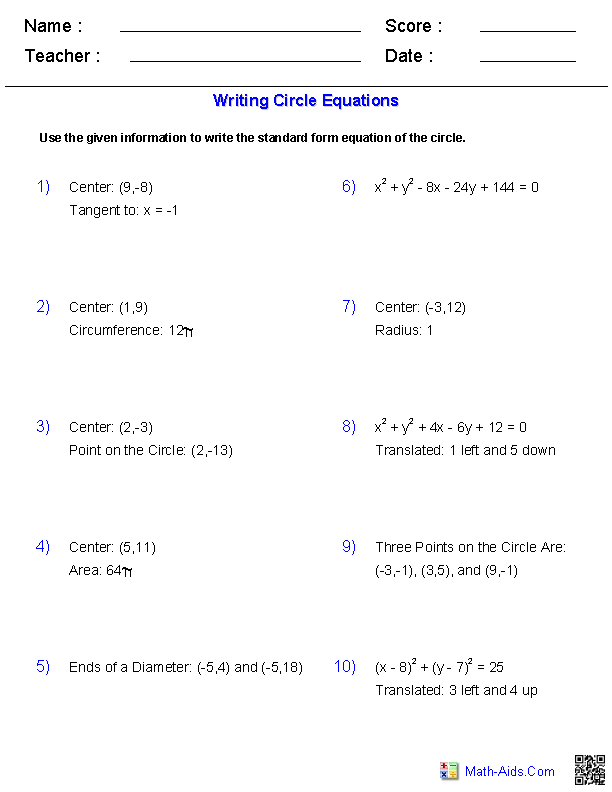##### Graphing Equationsof CirclesAlgebra 2 Worksheets##### Propertiesof EllipsesAlgebra 2 Worksheets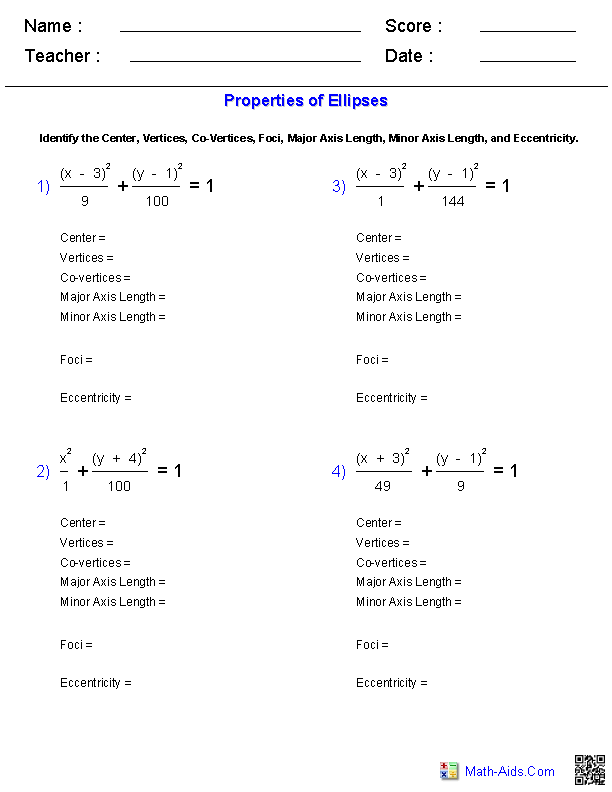##### Writing Equationsof EllipsesAlgebra 2 Worksheets##### Graphing Equationsof EllipsesAlgebra 2 Worksheets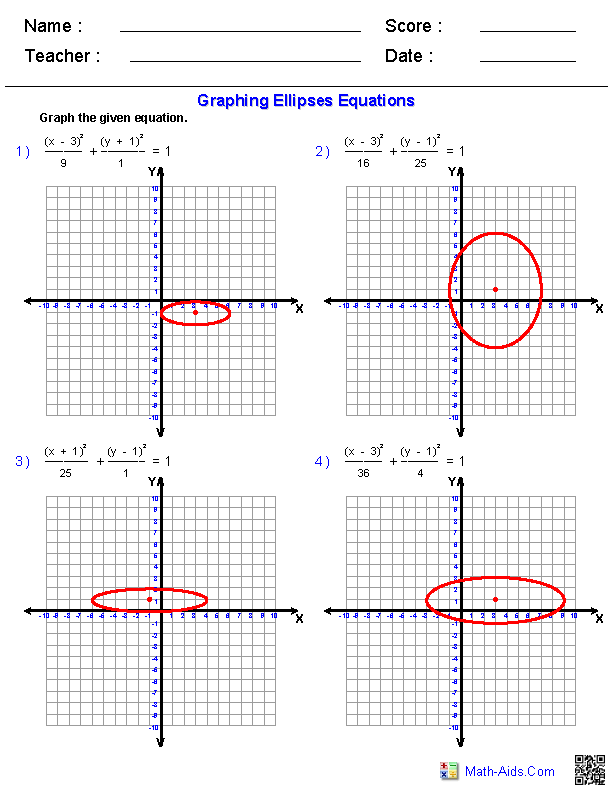##### Propertiesof ParabolasAlgebra 2 Worksheets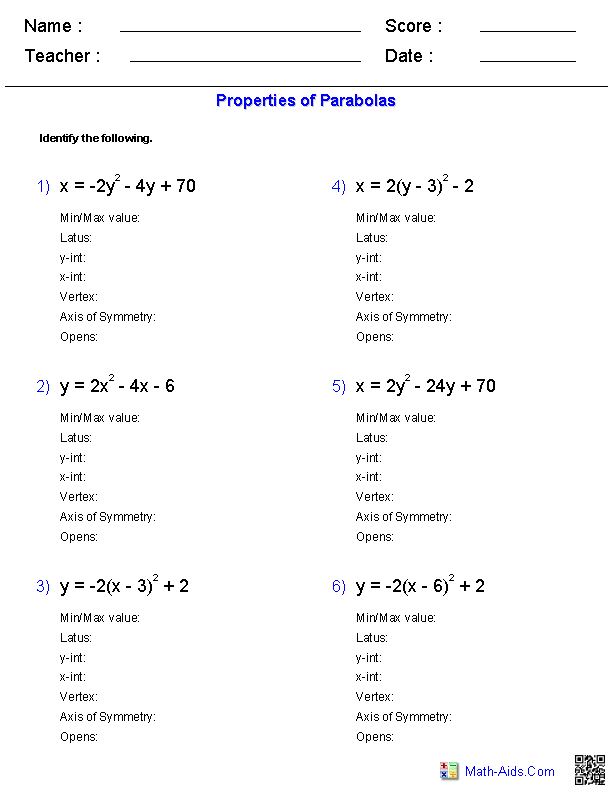##### Writing Equationsof ParabolasAlgebra 2 Worksheets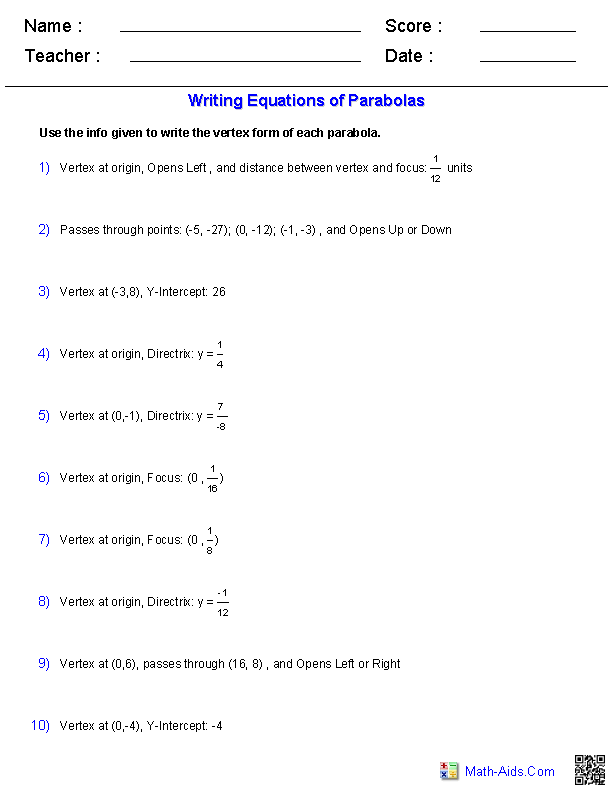##### Graphing Equationsof ParabolasAlgebra 2 Worksheets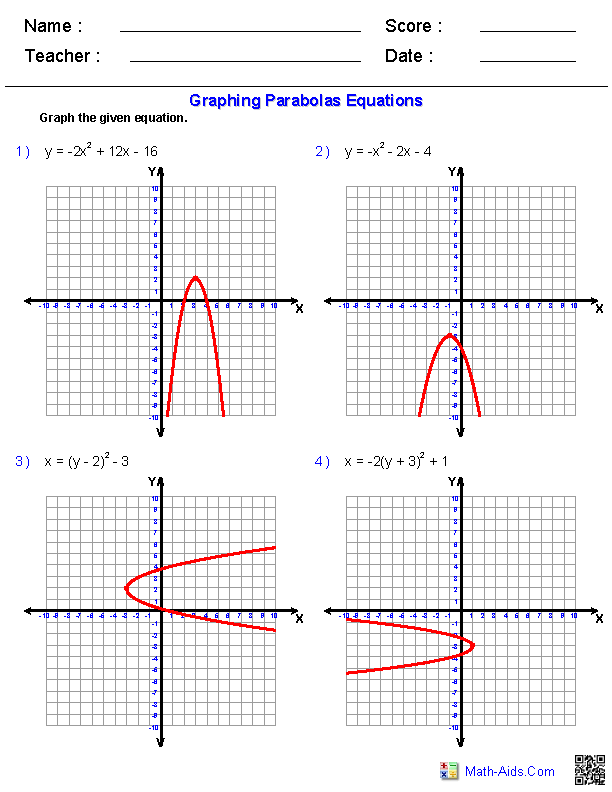##### Propertiesof HyperbolasAlgebra 2 Worksheets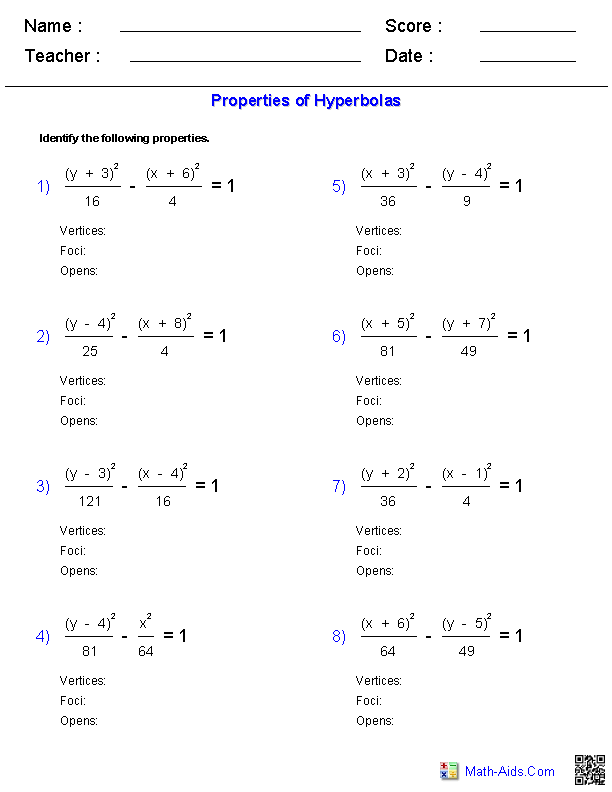##### Writing Equationsof HyperbolasAlgebra 2 Worksheets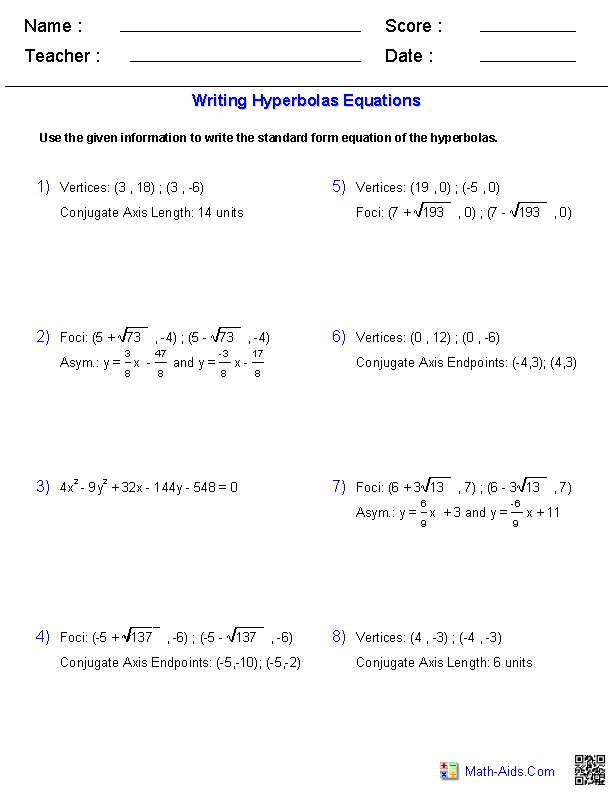##### Graphing Equationsof HyperbolasAlgebra 2 Worksheets##### Classifying Conic SectionsAlgebra 2 Worksheets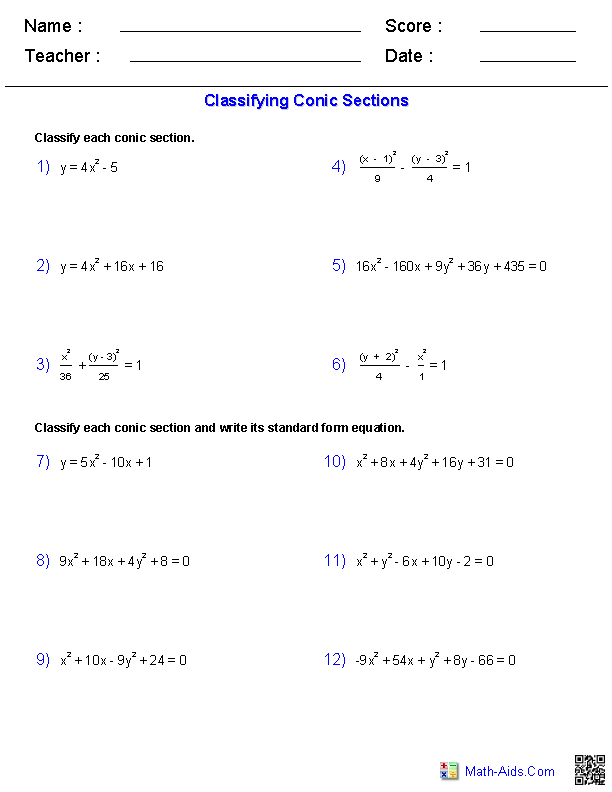##### Eccentricity of aConic Sections Worksheets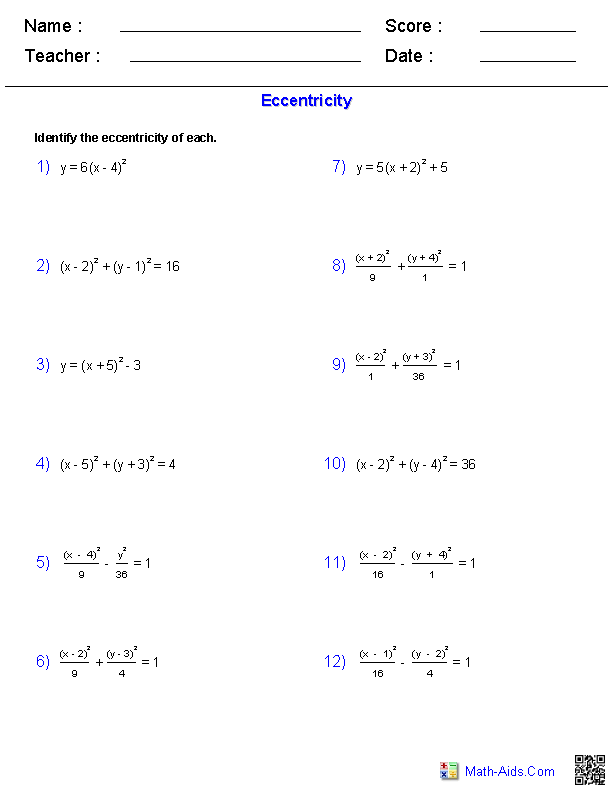Recommended Videos

## Detailed Description for All Conic Sections Worksheets

Properties of Circles Worksheets
These Conic Sections Worksheets will produce problems for the student to determine the center and radius from a given equation. You may select which types of numbers will be used in the problems as well as the form of the equations. These Conic Sections Worksheets are a good resource for students in the 8th Grade through the 12th Grade.

Writing Equations of Circles Worksheets
These Conic Sections Worksheets will produce problems for writing equations of circles. You may select which types of problems to use. These Conic Sections Worksheets are a good resource for students in the 8th Grade through the 12th Grade.

Graphing Equations of Circles Worksheets
These Conic Sections Worksheets will produce problems for practicing graphing circles from their equations. These Conic Sections Worksheets are a good resource for students in the 8th Grade through the 12th Grade.

Properties of Ellipses Worksheets
These Conic Sections Worksheets will produce problems for properties of ellipses. You may select which properties to identify. These Conic Sections Worksheets are a good resource for students in the 8th Grade through the 12th Grade.

Writing Equations of Ellipses Worksheets
These Conic Sections Worksheets will produce problems for writing equations of ellipses. You may select the ellipses properties given to write the equation. These Conic Sections Worksheets are a good resource for students in the 8th Grade through the 12th Grade.

Graphing Equations of Ellipses Worksheets
These Conic Sections Worksheets will produce problems for practicing graphing Ellipses from their equations. These Conic Sections Worksheets are a good resource for students in the 8th Grade through the 12th Grade.

Properties of Parabolas Worksheets
These Conic Sections Worksheets will produce problems for properties of parabolas. You may select which properties to identify, and in what form the equations will be. These Conic Sections Worksheets are a good resource for students in the 8th Grade through the 12th Grade.

Writing Equations of Parabolas Worksheets
These Conic Sections Worksheets will produce problems for writing equations of parabolas. You may select the parabolas properties given to write the equation. These Conic Sections Worksheets are a good resource for students in the 8th Grade through the 12th Grade.

Graphing Equations of Parabolas Worksheets
These Conic Sections Worksheets will produce problems for practicing graphing Parabolas from their equations. These Conic Sections Worksheets are a good resource for students in the 8th Grade through the 12th Grade.

Properties of Hyperbolas Worksheets
These Conic Sections Worksheets will produce problems for properties of hyperbolas. You may select which properties to identify. These Conic Sections Worksheets are a good resource for students in the 8th Grade through the 12th Grade.

Writing Equations of Hyperbolas Worksheets
These Conic Sections Worksheets will produce problems for writing equations of hyperbolas. You may select the hyperbolas properties given to write the equation. These Conic Sections Worksheets are a good resource for students in the 8th Grade through the 12th Grade.

Graphing Equations of Hyperbolas Worksheets
These Conic Sections Worksheets will produce problems for practicing graphing hyperbolas from their equations. These Conic Sections Worksheets are a good resource for students in the 8th Grade through the 12th Grade.

Classifying Conic Sections Worksheets
These Conic Sections Worksheets will produce problems for classifying conic sections. You may select which type of conic sections to use in the problems. These Conic Sections Worksheets are a good resource for students in the 8th Grade through the 12th Grade.

Eccentricity Worksheets
These Conic Sections Worksheets will produce problems for eccentricity. You may select which type of conic sections to use in the problems. These Conic Sections Worksheets are a good resource for students in the 8th Grade through the 12th Grade.